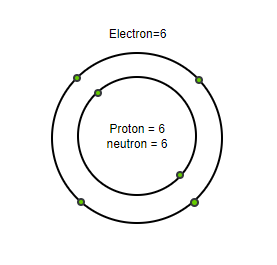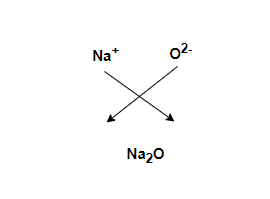Open in App
Not now

## Related Articles

• CBSE Class 9 Syllabus (All Subjects)
• CBSE Class 9 Maths Notes
• What is Vaporization?
• What is the Significance of the Formula of a Substance?

# What is the Significance of the Formula of a Substance?

• Last Updated : 31 Dec, 2021

Everyone may think that if the atom is very tiny in size, what is its importance of it? So, this is because the entire world is made up of atoms and molecules. As known that one cannot see them with the naked eyes but they are always present around. They may be in the surrounding, substances that everybody uses, or even in our own human body. They are constantly affecting whatever done. Luckily, though some scientific studies and technologies, now some magnified images of the surfaces of substances showing atoms and molecules are clearer and more understood.

### Atoms

Atoms can be defined as the tiny substances present in an element or compound which can take part in chemical reactions. An atom is made up of three major parts: protons, neutrons, and electrons.

• Protons: The positively charged particles in an atom is called proton.
• Neutrons: The chargeless particles in an atom is called a neutron.
• Electrons: The negatively charged particles in an atom is called electron.

As known that, most of the atoms are usually stable in nature. But some of the atoms have too many neutrons in their nucleus, which makes them unstable. A human body is made up of 99 percent of atoms of carbon, hydrogen, nitrogen, and oxygen. Below is an example of an atomic structure for your reference:### Atomic mass

The atomic mass of an element is the average mass of the atoms which can be measured in the atomic mass unit [amu]. The formula to calculate the atomic mass unit is,

atomic mass = number of protons + number of neutrons

Example: Calculate the atomic mass of potassium?

Number of protons = 19

Number of neutrons = 20

Now,

Atomic mass = number of protons + number of neutrons

= 19 + 2

= 39 a.m.u

### Compound

A compound can be defined as a substance that is made by the combination of two or more elements. Some of the examples of compounds are:

• Calcium hydroxide
• Sulphuric acid
• Boric acid
• Acetic acid
• Ammonia
• Alcohol

## Molecules

A molecule can be defined as the electrically neutral group of atoms formed together by chemical bonds. Molecules are made up of two or more two atoms. The atoms can be of the same element or from different elements in different situations. Some of the examples of molecules are:

1. Water
2. Carbon dioxide
3. Glucose
4. Ozone
5. Sodium chloride

Molecular formula

A molecular formula is defined as the chemical formula which gives us the total number of atoms of each element present in the molecule of an object.

Molecular mass

The total mass of a molecule of a substance is called molecular mass. It is measured by daltons. The formula to calculate the molecular mass is,

molecular = atomic weight × number of atoms

Example: Calculate the molecular mass of water?

Mass of hydrogen = 1

Mass of oxygen = 16

Number of atoms =3

Now,

Molecular mass = atomic weight × number of atoms

= 2 × mass of now hydrogen atom + mass of one oxygen atom

= 2 × 1 +16

= 18 u

Molecular structure

The molecular structure is the figure of a molecule that explains the location of atoms. One example is given below for your reference,Molecular structure of sodium oxide

### Significance of the formula of a substance

So far, it is seen that chemical formula for different elements and molecules exist, but why do they exist? what is significance of the formula of a substance. Below given are some essential points that explains the significance of the formula of any substance.

• A chemical formula represents the name of a substance.
• Chemical formulas help us to provide important information about the chemical formation of an element or compound.
• It also helps us to recognize the total number of atoms of an element and molecules of a particular substance.
• It gives a definite mass of an object.
• It represents one mole of molecules of a substance.
• They help us to write a chemical reaction and its progress in a short period of time.

### Sample Problems

Question 1: What is the atomic mass. What are atoms made up of?

The total mass of an atom is known as atomic mass. Atom is made up of proton, neutron, and electron.

Question 2: Calculate the atomic mass of potassium.

Solution:

The atomic mass of potassium is,

Number of protons = 19

Number of neutrons = 20

Now,

Atomic mass = number of protons + number of neutrons

= 19 + 20

= 39 a.m.u

Question 3: How atoms and molecules are interrelated?

Molecules are substances that are made up of two or more atoms in an element. So, if atoms don’t exist the existence of molecules is also impossible.

Question 4: What is molecular mass? Calculate the molecular mass of carbon dioxide.

Solution:

The total mass of a molecular is known as molecular mass. The molecular mass of carbon dioxide is,

Mass of carbon atom = 6

Mass of one oxygen atom = 8

Now,

molecular mass = 6 + 8 × 2

= 6 + 16

= 22 μ.

Question 5: Mention any two significance of formula of a substance.